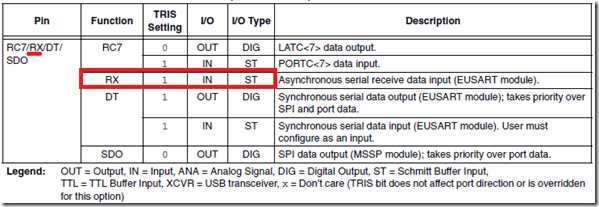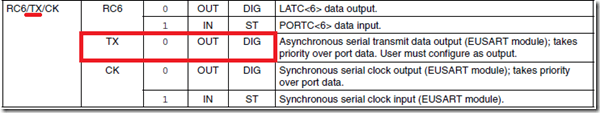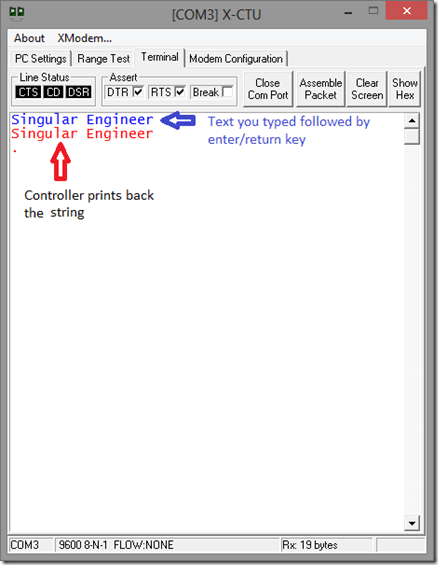# Tag: XC8 compiler

## Programming PIC 18 using XC8 (MPLAB X) : USART (PIC18F Serial)I was about to start Analog to Digital Converters (ADC), but then how am I going to see the output? We need some type of debug terminal and so decided to start with serial port or say Universal Synchronous/Asynchronous Receiver/Transmitter. Serial communication is one of the best debug tool ever when it comes to embedded systems. In order to know what’s going on inside the controller and how your firmware is behaving, serial port serves as a window to look into.

So first we need to set the speed/baud rate and here is the formulaWhat’s the difference between Asynchronous and Synchronous?

Asynchronous = Transmit (TX) and Receive (RX) can happen at same time – full duplex

Synchronous = Either TX or RX at a time – half duplex

Ok, so now I’m going to set my UART (asynchronous and high speed – BRGH = 1) to 9600bauds. My controller is running at 8MHz internal oscillator. So according to the formula

16(X+1) = Fosc/Baud Rate

16(X+1) = 8000000/9600

16(X+1) = 833.333

X+ 1 = 833.333/16

X+1 = 52.0833

X = 51.0833

X = 51

So my settings is going to be: SPBRG = 51, BRGH = 1, Asynchronous mode

Setting the USART PinsSetting the RX and TX pins according to the table given above (you can find the table in the datasheet of the device you are using).

```    TRISCbits.RC6 = 0; //TX pin set as output
TRISCbits.RC7 = 1; //RX pin set as input```

Read the peripheral library for function definitions before blindly copying the code.

 Note: If the PIC has more than 1 UART peripheral then you use the following Open_nUSART() puts_nUSART() write_nUSART() Where n = UART number E.g.: Open_1USART() puts_1USART() etc.,
```#define _XTAL_FREQ 8000000 //The speed of your internal(or)external oscillator
#include <xc.h>
#include "config.h"
#include <plib/usart.h>
int i = 0;
unsigned char UART1Config = 0, baud = 0;
unsigned char MsgFromPIC[] = "PIC Rocks\r";

void SetupClock(void);
void Delay1Second(void);

void main(int argc, char** argv) {

SetupClock(); // Internal Clock to 8MHz

TRISCbits.RC6 = 0; //TX pin set as output
TRISCbits.RC7 = 1; //RX pin set as input

UART1Config = USART_TX_INT_OFF & USART_RX_INT_OFF & USART_ASYNCH_MODE & USART_EIGHT_BIT & USART_BRGH_HIGH ;
baud = 51;
OpenUSART(UART1Config,baud);

while(1) //infinite loop
{
putsUSART(MsgFromPIC);
Delay1Second();
}
}

void SetupClock()
{
OSCCONbits.IRCF0 = 1;
OSCCONbits.IRCF1 = 1;
OSCCONbits.IRCF2 = 1;
}

void Delay1Second()
{
for(i=0;i<100;i++)
{
__delay_ms(10);
}
}```

The code above will print “PIC Rocks” every one second. Now, that is the communication from PIC to outside. Next will be to make PIC receive what you send in. Please read the peripheral library document located at “C:\Program Files (x86)\Microchip\xc8\<version>\docs\pic18_plib.pdf”

Now to receive from serial pin you can do it 2 ways.

• Polling
• Interrupts

Polling is something you will find everywhere so I’m not going to show here. But interrupts on the other way is the most efficient way to handle serial incoming data by a controller.

To enable interrupt change USART_RX_INT_OFF to USART_RX_INT_ON and then  enable the peripheral interrupt. In the data sheet you’ll find this table from which you can set the interrupt bitsUART receive in PIC might not receive [OR] RX pin might not work if you don’t read the note below

 Note: Most RX pins are shared with ADC. Please disable the ADC using ANSELC (or corresponding ANSEL) eg: ANSELC = 0x00 will make all pins as DIO in port C.

Here is the code for 1 character echo program

```#define _XTAL_FREQ 8000000 //The speed of your internal(or)external oscillator
#include <xc.h>
#include "config.h"
#include <plib/usart.h>

unsigned char UART1Config = 0, baud = 0;
unsigned char MsgFromPIC[] = "\r\nYou typed :";
char rx;
void SetupClock(void);
void Delay1Second(void);

void main(int argc, char** argv) {

SetupClock(); // Internal Clock to 8MHz

TRISCbits.RC6 = 0; //TX pin set as output
TRISCbits.RC7 = 1; //RX pin set as input

UART1Config = USART_TX_INT_OFF & USART_RX_INT_ON & USART_ASYNCH_MODE & USART_EIGHT_BIT & USART_BRGH_HIGH ;
baud = 51;
OpenUSART(UART1Config,baud);

//compare with the table above
RCIF = 0; //reset RX pin flag
RCIP = 0; //Not high priority
RCIE = 1; //Enable RX interrupt
PEIE = 1; //Enable pheripheral interrupt (serial port is a pheripheral)

ei();       //remember the master switch for interrupt?

while(1) //infinite loop
{

}
}

void SetupClock()
{
OSCCONbits.IRCF0 = 1;
OSCCONbits.IRCF1 = 1;
OSCCONbits.IRCF2 = 1;
}

void interrupt SerialRxPinInterrupt()
{
//check if the interrupt is caused by RX pin
if(PIR1bits.RCIF == 1)
{
putsUSART(MsgFromPIC);
WriteUSART(rx);
PIR1bits.RCIF = 0; // clear rx flag
}

}```

Here is a program that will repeat what you typed when you hit the return (enter) key. The way the program works is, it will receive all the characters you type in. As it stores into an array, it will also look for return key / carriage return (which is 0x0D in hex).

```/*
* File:   main.c
* Author: Singular Engineer
*
* Created on May 22, 2013, 8:31 AM
*/

#define _XTAL_FREQ 8000000 //The speed of your internal(or)external oscillator

#include <xc.h>
#include "config.h"
#include <plib/usart.h>

unsigned char UART1Config = 0, baud = 0;
unsigned char MsgFromPIC[] = "\r\nYou typed :";
unsigned char MessageBuffer;

int i=0;
void SetupClock(void);
void Delay1Second(void);

void main(int argc, char** argv) {

SetupClock(); // Internal Clock to 8MHz

TRISCbits.RC6 = 0; //TX pin set as output
TRISCbits.RC7 = 1; //RX pin set as input

UART1Config = USART_TX_INT_OFF & USART_RX_INT_ON & USART_ASYNCH_MODE & USART_EIGHT_BIT & USART_BRGH_HIGH ;
baud = 51;
OpenUSART(UART1Config,baud);

//Compare with the table above
RCIF = 0; //reset RX pin flag
RCIP = 0; //Not high priority
RCIE = 1; //Enable RX interrupt
PEIE = 1; //Enable pheripheral interrupt (serial port is a pheripheral)

ei();       //remember the master switch for interrupt?

while(1) //infinite loop
{

}
}

void SetupClock()
{
OSCCONbits.IRCF0 = 1;
OSCCONbits.IRCF1 = 1;
OSCCONbits.IRCF2 = 1;
}

void interrupt SerialRxPinInterrupt()
{
//check if the interrupt is caused by RX pin
if(PIR1bits.RCIF == 1)
{
if(i<200) //our buffer size
{
if(MessageBuffer[i] == 0x0D) //check for return key
{
putsUSART(MessageBuffer);
for(;i>0;i--)
MessageBuffer[i] = 0x00; //clear the array
i=0; //for sanity
return;
}
i++;
PIR1bits.RCIF = 0; // clear rx flag
}
else
{
putsUSART(MessageBuffer);
for(;i>0;i--)
MessageBuffer[i] = 0x00; //clear the array
i=0; //for sanity
return;
}
}
}```

And here is the output